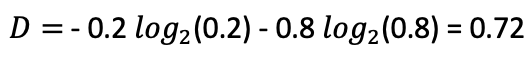top of page
Search
•Sai Geetha M N

# Decision Trees - Homogeneity Measures

Having had an introduction to what is homogeneity and what are the 3 basic types of measures that can be used in the previous article on "Decision Trees: How to Decide the split?", today we will look at each of the measures in detail with examples, to get an intuitive understanding of what these measures are trying to measure. (Whoa! A mouthful of measure here :))

Just to recap, I said that the most often used measures of impurity are

1. Gini Index

2. Entropy

The other measure called the Classification Error is hardly ever used but helps us in getting an understanding of how impurity is measured and hence I will explain that too, here.

## Classification Error

This is the error we see when we go with a simplistic method of assigning the majority class label to a node. It means all of the minority data points are misclassified. The probability of misclassification of the minority points is taken as the classification error. Very Simple!

Let us take an example:

Assume we have a dataset with 1000 points. 200 of them belong to Class A and 800 of them belong to Class B, as shown here:This dataset is neither clearly impure nor clearly pure. It seems to have a majority of Class B data. So, to check the purity of this node, we take the probability of a point belonging to either class A or class B

The probability of a data point belonging to class A in this data set can be written as :And the probability of a data point belonging to class B can be written as:So, now if we assign the majority class label to this node, it would get the label of Class B. This would imply that 20% of the points (belonging to Class A) are misclassified. They actually belong to class A but are classified as class B. Hence, the probability of the minority class becomes the error rate. Therefore, the classification error E is defined as:where p_max is the probability of the majority class.

This should intuitively explain what we are trying to call a classification error. The probability of the minority class itself is the error rate. It is sometimes also called the misclassification measure.

This should lay the intuitive basis for understanding the measure we use to decide the impurity or purity of a node

Let's move on to Gini Index

## Gini Index

Gini index is defined as the sum of p(1-p) over all classes where p is the probability of each class and is represented better as:where i runs from 1 to K - the number of classes in the data.

So, if we take the same example for which we calculated the classification errors, the Gini index would be:For each class, we calculate p(1-p) and add them up to get the Gini Index.

We see that while the classification error value was 0.2, the Gini Index is 0.32. It is a bit harsher on the misclassification and rightfully so. i.e. it penalizes the misclassification a little more harshly.

Now let us look at Entropy

## Entropy

This concept has come from Physics - in particular thermodynamics where entropy is defined as a measure of randomness or disorder of a system. And in some sense that is what we are also trying to measure when we look for impurity.

Here, it is defined as the sum of p*logp (log to the base 2) where p is the probability of each class in that dataset. It is represented as:If we apply this formula to the same example above, here's what we get:Here, the score seems, even more, harsher than Gini Index. But when scaled equally, they almost penalize very similarly to each other, which we will understand later.

To understand how these three measures compare to each other and what they convey as well, we will have to go with a few more cases of impurity combinations in order to plot a graph for each of them and understand them.

### Case of Maximum Impurity

Let us take the case when there is an equal number of data points from 2 different classes in a data node. i.e. 50% each.

If we take the probability of both the classes as 0.5 and apply the three formulae, we get the following values:

Classification error = 0.5

Gini Impurity = 0.5

Entropy = 1

These are the maximum impurity values that each can take on. The minimum is 0 in all the cases.

To take this further, I have taken the following dataset of class distribution variation and plotted a graph.Starting from a pure dataset with only Class B data points and then going on increasing the impurity till both the classes are 50 each and finally reversing the impurity of the classes, the data you get for all three measures is shown here.

If we were to plot this data onto a graph, what you find is this:We see that the graphs are symmetric in nature, as expected. It does not matter which class is the majority, the impurity is the same, even if the numbers are swapped between the classes. Here log (0) is not taken as undefined but approximated to 0 for the Entropy function.

Notice the obvious symmetry and the fact that the classification error is linear, raises much slower than the other two, and falls similarly. Entropy and Gini are more rapid in the rise and fall and are actually very similar to each other if entropy was scaled down to Gini levels.

In practice, either of them can be used in terms of the measurement of impurity. However, computing power requirements may tilt many to use Gini more often.

Hope you enjoyed this. I would look forward to hearing any feedback or comments.